Скачать презентацию Learning with Positive and Unlabeled Examples using Weighted

8d7213cd0f79d524178b23f4c65550d0.ppt

• Количество слайдов: 15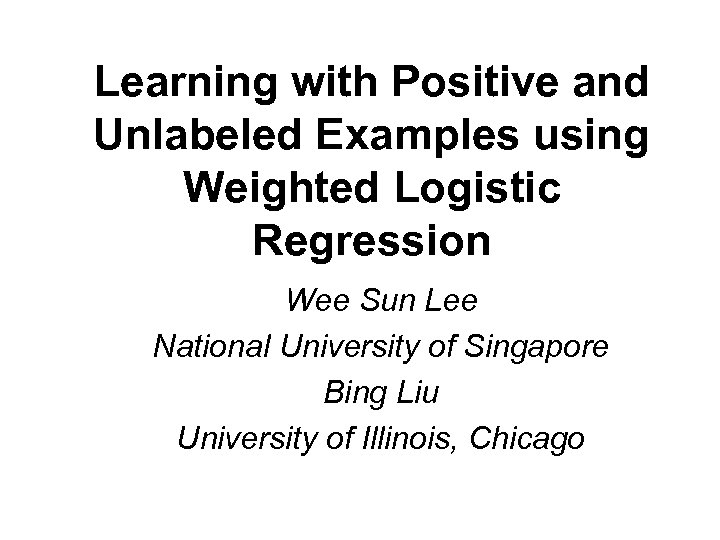Learning with Positive and Unlabeled Examples using Weighted Logistic Regression Wee Sun Lee National University of Singapore Bing Liu University of Illinois, Chicago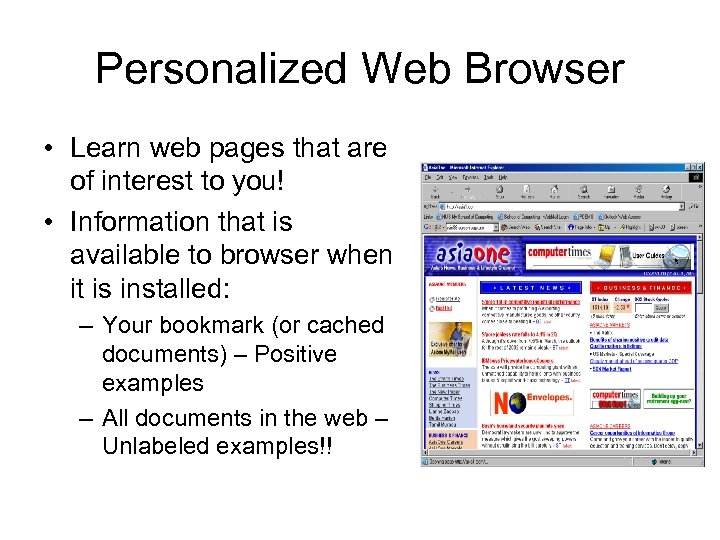Personalized Web Browser • Learn web pages that are of interest to you! • Information that is available to browser when it is installed: – Your bookmark (or cached documents) – Positive examples – All documents in the web – Unlabeled examples!!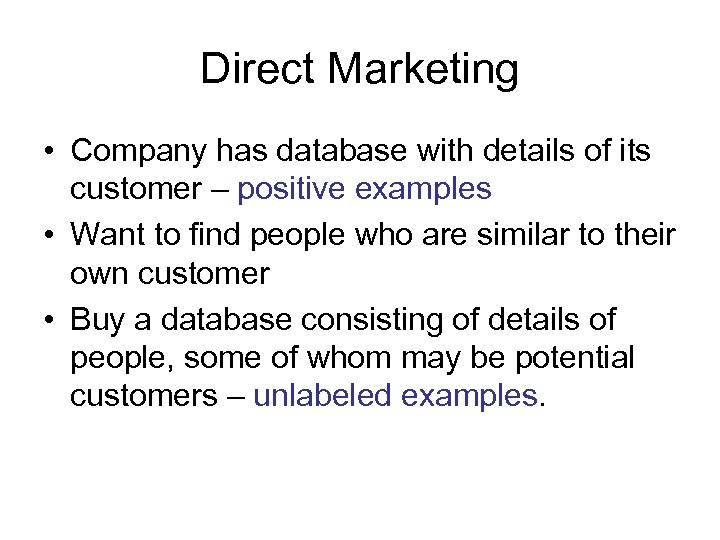Direct Marketing • Company has database with details of its customer – positive examples • Want to find people who are similar to their own customer • Buy a database consisting of details of people, some of whom may be potential customers – unlabeled examples.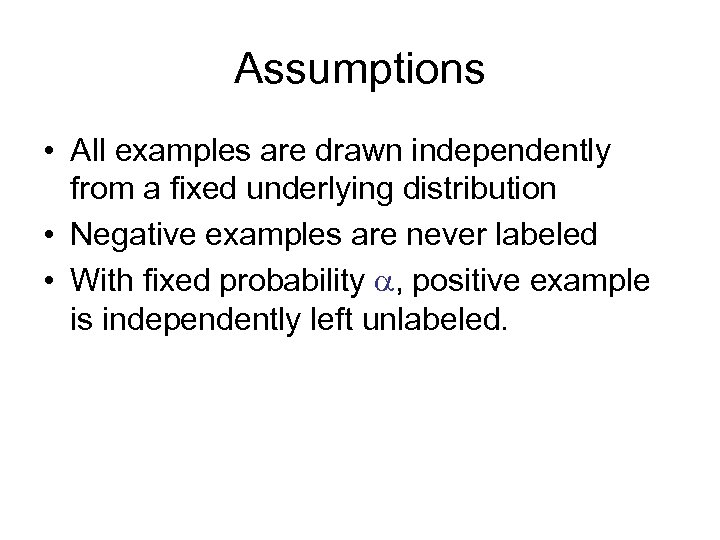Assumptions • All examples are drawn independently from a fixed underlying distribution • Negative examples are never labeled • With fixed probability , positive example is independently left unlabeled.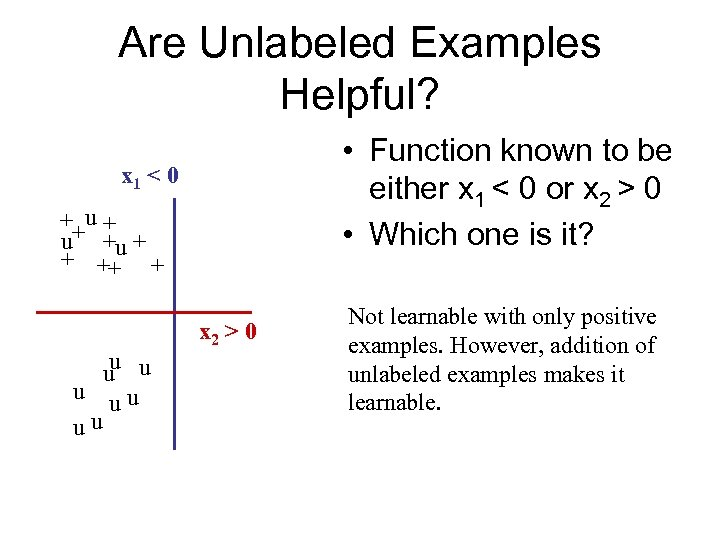Are Unlabeled Examples Helpful? • Function known to be either x 1 < 0 or x 2 > 0 • Which one is it? x 1 < 0 ++u + u +u + + ++ + u u uu uu x 2 > 0 Not learnable with only positive examples. However, addition of unlabeled examples makes it learnable.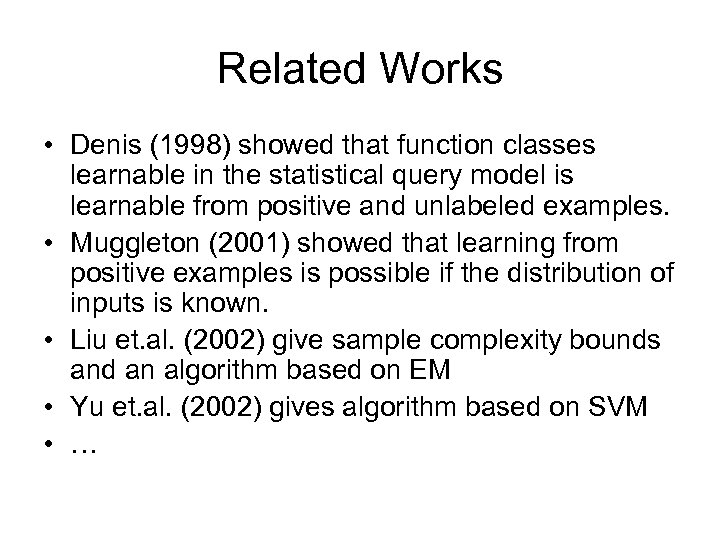Related Works • Denis (1998) showed that function classes learnable in the statistical query model is learnable from positive and unlabeled examples. • Muggleton (2001) showed that learning from positive examples is possible if the distribution of inputs is known. • Liu et. al. (2002) give sample complexity bounds and an algorithm based on EM • Yu et. al. (2002) gives algorithm based on SVM • …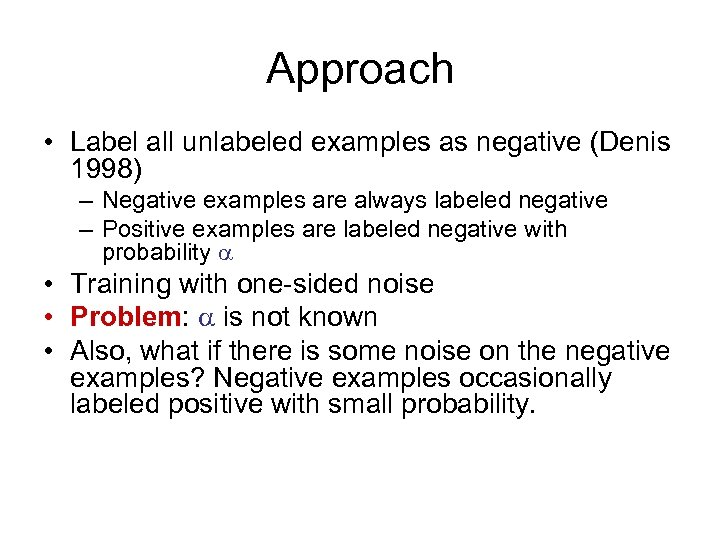Approach • Label all unlabeled examples as negative (Denis 1998) – Negative examples are always labeled negative – Positive examples are labeled negative with probability • Training with one-sided noise • Problem: is not known • Also, what if there is some noise on the negative examples? Negative examples occasionally labeled positive with small probability.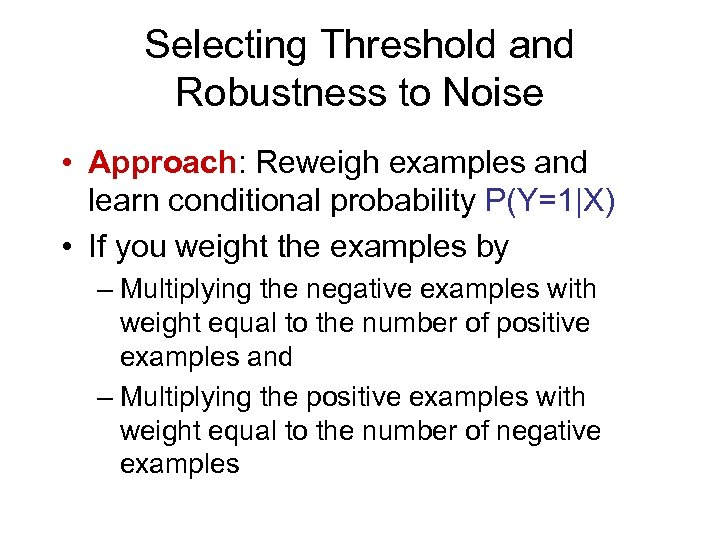Selecting Threshold and Robustness to Noise • Approach: Reweigh examples and learn conditional probability P(Y=1|X) • If you weight the examples by – Multiplying the negative examples with weight equal to the number of positive examples and – Multiplying the positive examples with weight equal to the number of negative examples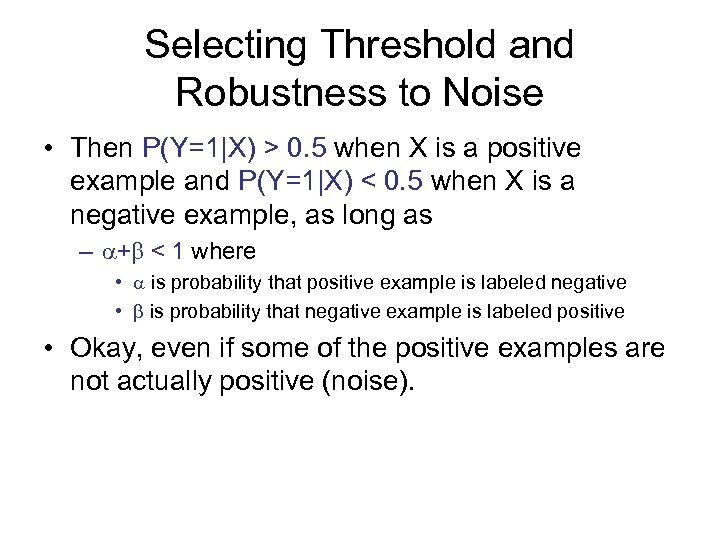Selecting Threshold and Robustness to Noise • Then P(Y=1|X) > 0. 5 when X is a positive example and P(Y=1|X) < 0. 5 when X is a negative example, as long as – + < 1 where • is probability that positive example is labeled negative • is probability that negative example is labeled positive • Okay, even if some of the positive examples are not actually positive (noise).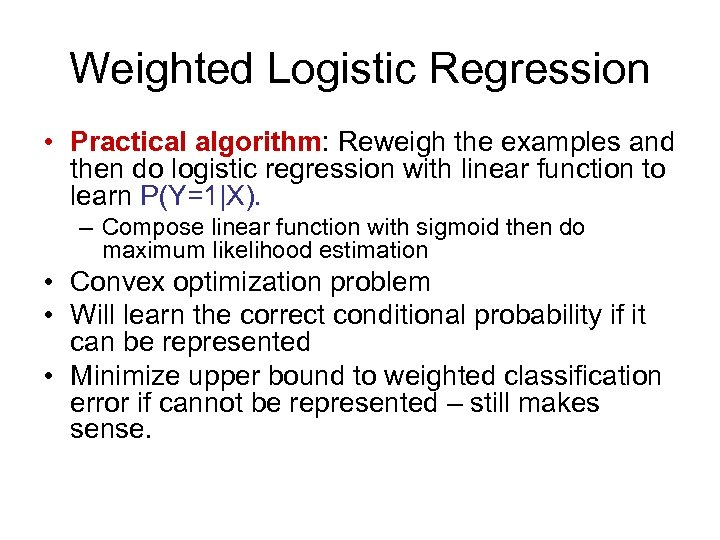Weighted Logistic Regression • Practical algorithm: Reweigh the examples and then do logistic regression with linear function to learn P(Y=1|X). – Compose linear function with sigmoid then do maximum likelihood estimation • Convex optimization problem • Will learn the correct conditional probability if it can be represented • Minimize upper bound to weighted classification error if cannot be represented – still makes sense.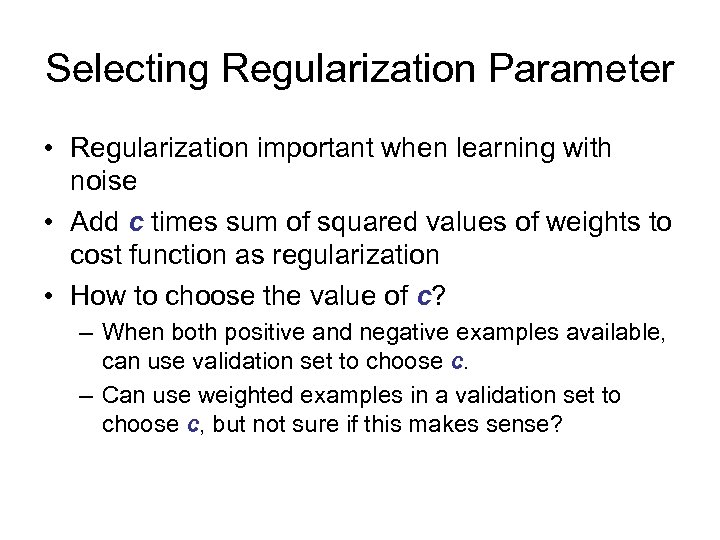Selecting Regularization Parameter • Regularization important when learning with noise • Add c times sum of squared values of weights to cost function as regularization • How to choose the value of c? – When both positive and negative examples available, can use validation set to choose c. – Can use weighted examples in a validation set to choose c, but not sure if this makes sense?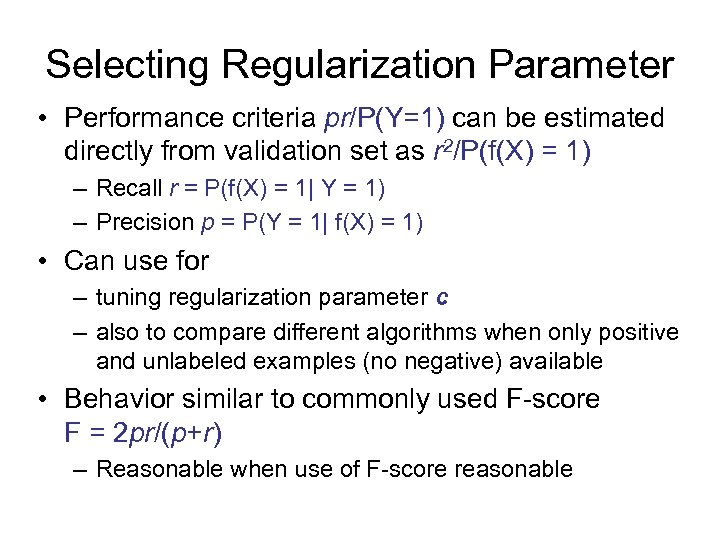Selecting Regularization Parameter • Performance criteria pr/P(Y=1) can be estimated directly from validation set as r 2/P(f(X) = 1) – Recall r = P(f(X) = 1| Y = 1) – Precision p = P(Y = 1| f(X) = 1) • Can use for – tuning regularization parameter c – also to compare different algorithms when only positive and unlabeled examples (no negative) available • Behavior similar to commonly used F-score F = 2 pr/(p+r) – Reasonable when use of F-score reasonable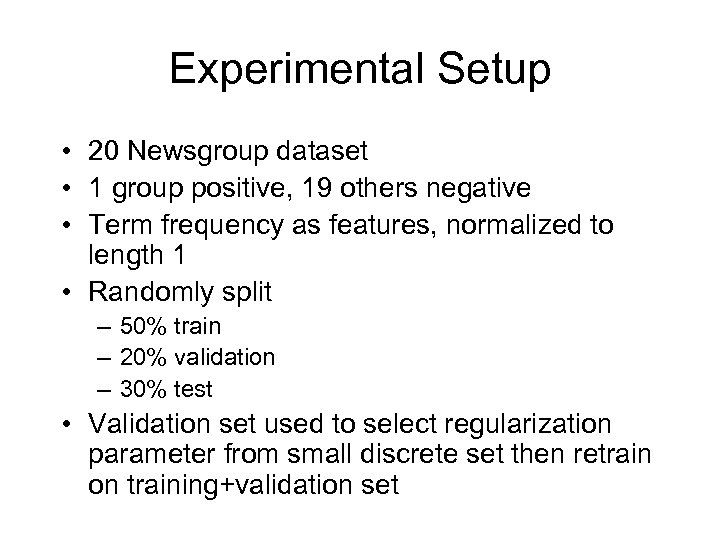Experimental Setup • 20 Newsgroup dataset • 1 group positive, 19 others negative • Term frequency as features, normalized to length 1 • Randomly split – 50% train – 20% validation – 30% test • Validation set used to select regularization parameter from small discrete set then retrain on training+validation set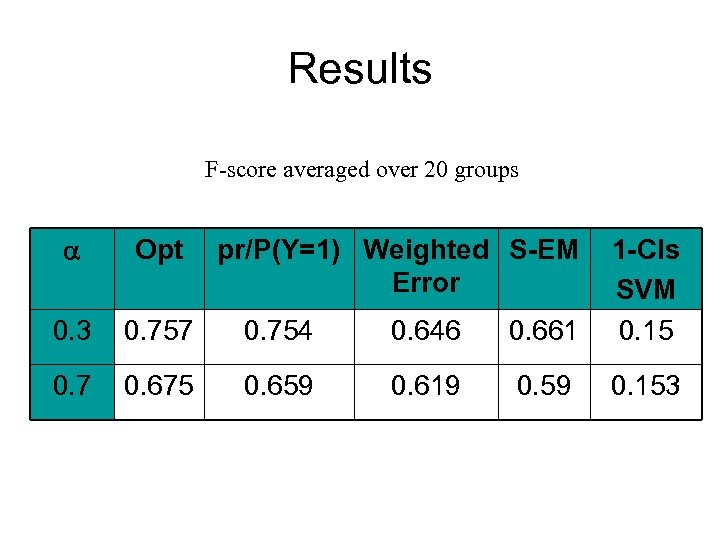Results F-score averaged over 20 groups Opt 0. 3 0. 757 0. 754 0. 646 0. 661 1 -Cls SVM 0. 15 0. 7 0. 675 0. 659 0. 619 0. 59 0. 153 pr/P(Y=1) Weighted S-EM Error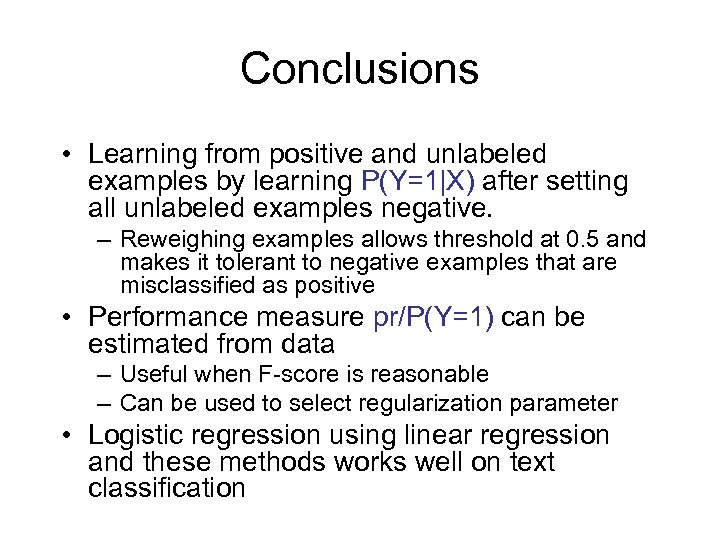Conclusions • Learning from positive and unlabeled examples by learning P(Y=1|X) after setting all unlabeled examples negative. – Reweighing examples allows threshold at 0. 5 and makes it tolerant to negative examples that are misclassified as positive • Performance measure pr/P(Y=1) can be estimated from data – Useful when F-score is reasonable – Can be used to select regularization parameter • Logistic regression using linear regression and these methods works well on text classification